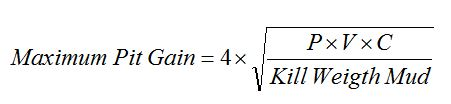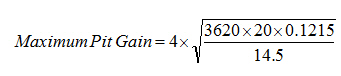## Maximum pit gain from gas kick in water based mud

In water based mud, you can not only estimate the maximum surface casing pressure, but you are also be able to determine the maximum pit gain due to gas influx.

The following formula demonstrates how to figure out the maximum pit gain from gas influx in water based mud system.Where

Maximum Pit Gain in bbl

P is formation pressure in psi.

V is original pit gain in bbl.

C is annular capacity in bbl/ft.

Kill Weight Mud in ppg

Let’s take a look at this following example in order to get more understanding regarding this topic.

Drill well with water based mud.

Pit gain = 20 bbl

Initial shut in casing pressure = 600 psi

Initial shut in drill pipe pressure = 500 psi

Current mud weight = 12.5 ppg

Hole depth = 6,000’MD/4,800’TVD

Hole diameter = 12-1/4 inch

Drill pipe = 5 inch

According to the data, you need to figure out the Kill Mud Weight , formation pressure, and annular capacity.

Kill Weight Mud = current mud weight + (shut in drill pipe pressure ÷ (0.052 x TVD))

Kill Weight Mud = 12.5 + (500 ÷ (0.052 x 4800)) = 14.5 ppg

Formation pressure = surface pressure + hydrostatic pressure

Formation pressure = 500 + (0.052 x 12.5 x 4800) = 3620 psi

Determine annular capacity: Annular capacity = (12.252 – 52) ÷ 1029.4 = 0.1215 bbl/ft

Note: if you would like to understand annular capacity, please check out the following links -> Annular Capacity

Once you get all parameters required, you can add all of them into the equation like this.Maximum Pit Gain = 98.5 bbl

References

Cormack, D. (2007). An introduction to well control calculations for drilling operations. 1st ed. Texas: Springer.

Crumpton, H. (2010). Well Control for Completions and Interventions. 1st ed. Texas: Gulf Publishing.

Grace, R. (2003). Blowout and well control handbook [recurso electrónico]. 1st ed. Paises Bajos: Gulf Professional Pub.